×#### Thank you for registering.

One of our academic counsellors will contact you within 1 working day.

Click to Chat

1800-1023-196

+91-120-4616500

CART 0

• 0

MY CART (5)

Use Coupon: CART20 and get 20% off on all online Study Material

ITEM
DETAILS
MRP
DISCOUNT
FINAL PRICE
Total Price: Rs.

There are no items in this cart.
Continue Shopping• Complete JEE Main/Advanced Course and Test Series
• OFFERED PRICE: Rs. 15,900
• View Details

```Chapter 5: Operations on Rational Numbers Exercise – 5.4

Question: 1

Divide: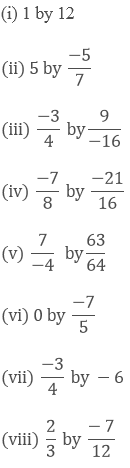Solution: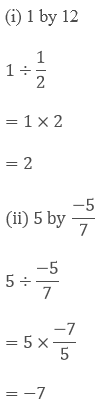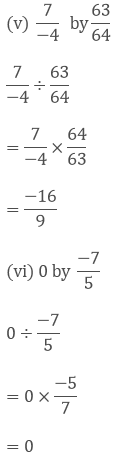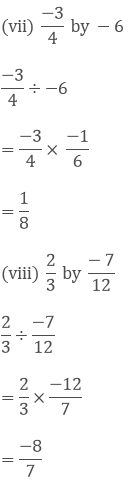Question: 2

Find the value and express as a rational number in standard form: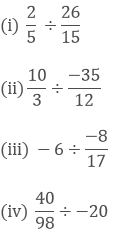Solution: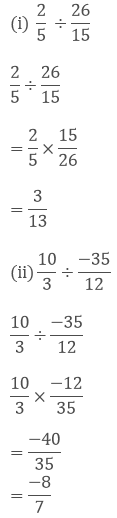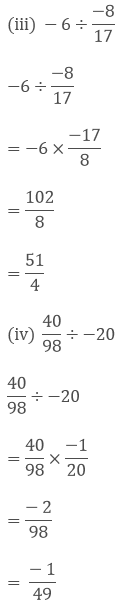Question: 3

The product of two rational numbers is 15. If one of the numbers is -10, find the other.

Solution:

Let the number to be found be x

x ×- 10 = 15

x = 15/(-10)

x = 3/(-2)

x = (-3)/2

Hence the number is x = (-3)/2

Question: 4

The product of two rational numbers is - 8/9. If one of the numbers is - 4/15, find the other.

Solution:

Let the number to be found be x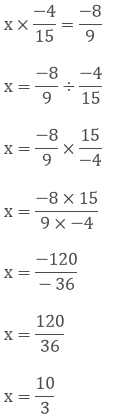Hence the number is x = 10/3

Question: 5

By what number should we multiply -1/6 so that the product may be -23/9?

Solution:

Let the number to be found be x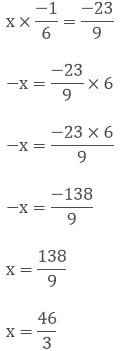Hence the number is x = 46/3

Question: 6

By what number should we multiply -15/28 so that the product may be -5/7?

Solution:

Let the number to be found be x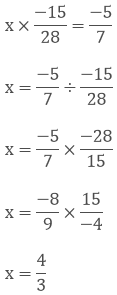Hence the number is x = 4/3

Question: 7

By what number should we multiply -8/13 so that the product may be 24?

Solution:

Let the number to be found be xx = - 39

Hence the number is x = - 39

Question: 8

By what number should -3/4 be multiplied in order to produce -2/3?

Solution:

Let the number to be found be xx = 8/9

Hence the number is x = 8/9

Question: 9

Find (x + y) ÷ (x —y), if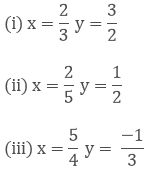Solution: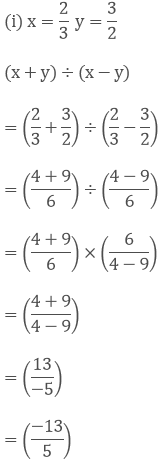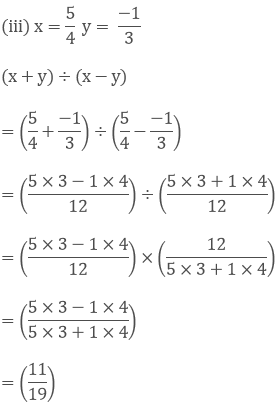Question: 10

The cost of 7(2/3) metres of rope is Rs. 12(3/4). Find its cost per metre. 7(2/3) metres of rope cost = Rs. 12(3/4).

Solution: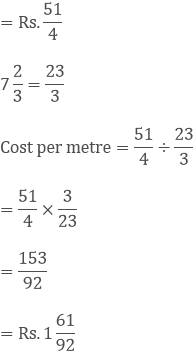Question: 11

The cost of 2(1/3) metres of cloth is Rs.75 1/4. Find the cost of cloth per metre. 2(1/3) metres of rope cost = Rs. 75(1/4)

Solution:Question: 12

By what number should (-33)/16 be divided to get (-11)/4?

Solution: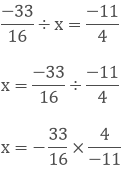x = 3/4

The number is x = 3/4

Question: 13

Divide the sum of (-13)/5 and 12/7 by the product of (-31)/7 and (-1)/2

Solution: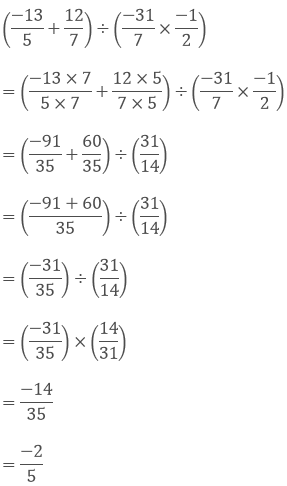Question: 14

Divide the sum of 65/12 and 8/3 by their difference.

Solution:Question: 15

If 24 trousers of equal size can be prepared in 54 metres of cloth, what length of cloth is required for each trouser?

Solution:= 54/24

= 9/4 metres

9/4 metres of cloth is required to make each trouser
```### Course Features

• 728 Video Lectures
• Revision Notes
• Previous Year Papers
• Mind Map
• Study Planner
• NCERT Solutions
• Discussion Forum
• Test paper with Video Solution